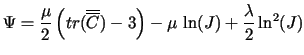Computational & Technology Resources
an online resource for computational,
engineering & technology publications
Civil-Comp Proceedings
ISSN 1759-3433
CCP: 75
PROCEEDINGS OF THE SIXTH INTERNATIONAL CONFERENCE ON COMPUTATIONAL STRUCTURES TECHNOLOGY
Edited by: B.H.V. Topping and Z. Bittnar
Paper 84

Numerical Solution by Energy Minimization of Hyperelastic Membrane in Large Displacements and Finite Strains

R. Bouzidi and A. Le Van

Laboratoire de Génie Civil de Nantes Saint-Nazaire, Faculty of Science and Technology, University of Nantes, France

Full Bibliographic Reference for this paper
R. Bouzidi, A. Le Van, "Numerical Solution by Energy Minimization of Hyperelastic Membrane in Large Displacements and Finite Strains", in B.H.V. Topping, Z. Bittnar, (Editors), "Proceedings of the Sixth International Conference on Computational Structures Technology", Civil-Comp Press, Stirlingshire, UK, Paper 84, 2002. doi:10.4203/ccp.75.84
Keywords: energy minimization, triangular finite element, circular membrane, rectangular membrane.

Summary
In this work, a numerical approach is presented for solving problems of finitely deformed membrane structures made of hyperelastic material and subjected to external pressure loadings. The problem is geometrically non-linear since it deals with finite displacements and rotations as well as finite strains. Furthermore, the material nonlinear behaviour is described by use of a hyperelastic constitutive relationship. Usually, the classical finite element method based on satisfaction of the equilibrium equations requires that geometric stiffness matrix and large displacement matrix are taken into account. Moreover, structure instabilities or bifurcations may occur under large displacements and material non-linearity, which must be predicted by a specific treatment. To correctly handle all the previous numerical problems, the classical finite element method usually leads to highly CPU time consuming programs.

In this paper, the membrane structures are solved by directly minimizing the total potential energy. Although theoretically equivalent to the traditional finite element method, the proposed approach proves to be a more robust and efficient technique. All the numerical computations are performed using a three-dimensional flexible triangular finite element, which is able to handle the abovementioned non-linearities. The finite element has no bending stiffness and the tangent matrix is singular for the rotation degrees of freedom. The numerical solution is carried out by means of an iterative method like the conjugate gradient or Newton one.

The strain energy is given by a quasi-incompressible hyperelastic potential density in the reference configuration written in the following form:(84.1)

First, the proposed finite element is validated through the well-known Hencky problem  which consists of the inflation of a circular membrane clamped at the rim. For this problem we recall the analytical solution in the case of isotropic linear elastic behavior [2,3], before the comparison between the analytical solution and the finite element results. Next, an extension to a nonlinear hyperelastic behavior is treated, which allows us to exhibit the pressure value which leads to the material instability of the circular membrane.

The second application is related to problem of an orthotropic rectangular membrane. We focus on two points: first we present a semi-analytical solution in the case of orthotropic linear behavior for the rectangular membrane. Then, this solution is used to validate once again the finite element in the case of isotropic behavior.

References
1
H. Hencky, "On the Stress State in Circular Plates With Vanishing Bending Stiffness", Zeitschrift f�r Mathematik und Physik, 63, 311-317, 1915.
2
W.B. Fichter, "Some solutions for the large deflections of uniformly loaded circular membranes", NASA Technical Paper 3658-NASA Langley Research Center, Hampton, VA, 1997.
3
J.D. Campbell, "On the theory of initially tensioned circular membranes subjected to uniform pressure", Quart J. Mech. & Appl. Math., Vol IC, Pt 1, 84-93, 1956.

purchase the full-text of this paper (price £20)